# How to number columns in Excel and convert column letter to number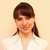by , updated on

The tutorial talks about how to return a column number in Excel using formulas and how to number columns automatically.

Last week, we discussed the most effective formulas to change column number to alphabet. If you have an opposite task to perform, below are the best techniques to convert a column name to number.

## How to return column number in Excel

To convert a column letter to column number in Excel, you can use this generic formula:

COLUMN(INDIRECT(letter&"1"))

For example, to get the number of column F, the formula is:

`=COLUMN(INDIRECT("F"&"1"))`

And here's how you can identify column numbers by letters input in predefined cells (A2 through A7 in our case):

`=COLUMN(INDIRECT(A2&"1"))`

Enter the above formula in B2, drag it down to the other cells in the column, and you will get this result: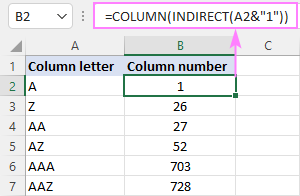How this formula works:

First, you construct a text string representing a cell reference. For this, you concatenate a letter and number 1. Then, you hand off the string to the INDIRECT function to convert it into an actual Excel reference. Finally, you pass the reference to the COLUMN function to get the column number.

## How to convert column letter to number (non-volatile formula)

Being a volatile function, INDIRECT can significantly slow down your Excel if used broadly in a workbook. To avoid this, you can identify the column number using a slightly more complex non-volatile alternative:

This works perfectly in dynamic array Excel (365 and 2021). In older version, you need to enter it as an array formula (Ctrl + Shift + Enter) to get it to work.

For example:

`=MATCH(A2&"1", ADDRESS(1, COLUMN(\$1:\$1), 4), 0)`

Or you can use this non-array formula in all Excel versions:

`=MATCH(A2&"1", INDEX(ADDRESS(1, INDEX(COLUMN(\$1:\$1), ), 4), ), 0)`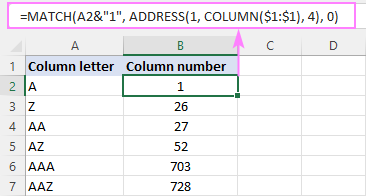How this formula works:

First off, you concatenate the letter in A2 and the row number "1" to construct a standard "A1" style reference. In this example, we have letter "A" in A2, so the resulting string is "A1".

Next, you get an array of strings representing all cell addresses in the first row, from "A1" to "XFD1". For this, you use the COLUMN(\$1:\$1) function, which generates a sequence of column numbers, and pass that array to the column_num argument of the ADDRESS function:

`ADDRESS(1, {1,2,3,4,5,…, 16384), 4)`

Given that row_num (1st argument) is set to 1 and abs_num (3rd argument) is set to 4 (meaning you want a relative reference), the ADDRESS function delivers this array:

`{"A1","B1","C1","D1",…,"XFD1"}`

Finally, you build a MATCH formula that searches for the concatenated string in the above array and returns the position of the found value, which corresponds to the column number you are looking for:

`MATCH("A1", {"A1","B1","C1","D1",…,"XFD1"}, 0)`

## Change column letter to number using a custom function

"Simplicity is the ultimate sophistication," stated the great artist and scientist Leonardo da Vinci. To get a column number from a letter in an easy way, you can create your own custom function.

Fully in line with the above principle, the function's code is as simple as it can possibly be:

Insert the code in your VBA editor as explained here, and your new function named ColumnNumber is ready for use.

The function requires just one argument, col_letter, which is the column letter to be converted into a number:

ColumnLetter(col_letter)

Your real formula can be as follows:

`=ColumnNumber(A2)`

If you compare the results returned by our custom function and Excel's native ones, you will make sure they are exactly the same: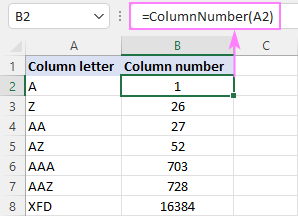## Return column number of a specific cell

To get a column number of a particular cell, simply use the COLUMN function:

For instance, to identify the column number of cell B3, the formula is:

`=COLUMN(B3)`

Obviously, the result is 2.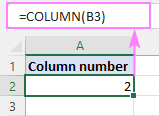## Get column letter of the current cell

To find out a column number of the current cell, use the COLUMN() function with an empty argument, so it refers to the cell where the formula is:

`=COLUMN()`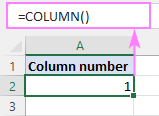## How to show column numbers in Excel

By default, Excel uses the A1 reference style and labels column headings with letters and rows with numbers. To get columns labeled with numbers, change the default reference style from A1 to R1C1. Here's how:

1. In your Excel, click File > Options.
2. In the Excel Options dialog box, select Formulas in the left pane.
3. Under Working with formulas, check the R1C1 reference style box, and click OK.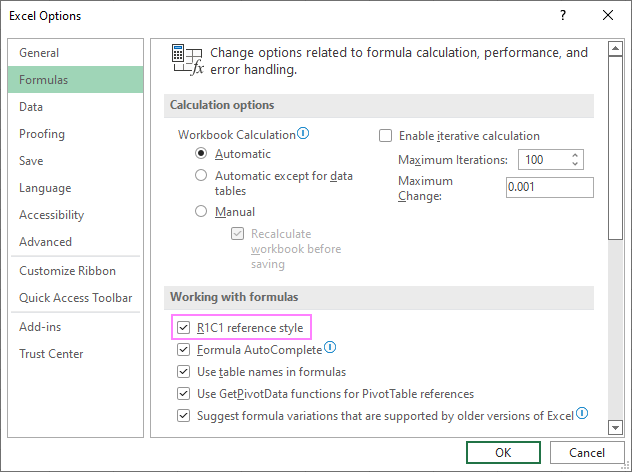The column labels will immediately change from letters to numbers: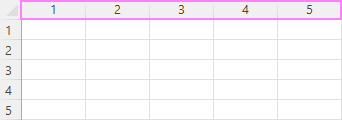Please note that selecting this option will not only change column labels - cell addresses will also change from A1 to R1C1 references, where R means "row" and C means "column". For example, R1C1 refers to the cell in row 1 column 1, which corresponds to the A1 reference. R2C3 refers to the cell in row 2 column 3, which corresponds to the C2 reference.

In existing formulas, cell references will update automatically, while in new formulas you will have to use the R1C1 reference style.

Tip. To revert back to A1 style, uncheck the R1C1 reference style check box in Excel Options.

## How to number columns in Excel

If you are not used to the R1C1 reference style and want to keep A1 references in your formulas, then you can insert numbers in the first row of our worksheet, so you have both - column letters and numbers. This can be easily done with the help of the Auto Fill feature.

Here are the detailed steps:

1. In A1, type number 1.
2. In B1, type number 2.
3. Select cells A1 and B1.
4. Hover the cursor over a small square in the lower right corner of cell B1, which is called the Fill handle. As you do this, the cursor will change to a thick black cross.
5. Drag the fill handle to the right up to the column you need.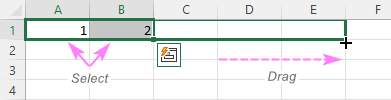As a result, you will retain the column labels as letters, and underneath the letters you will have the column numbers.

Tip. To keep the columns numbers in view while scrolling to the below areas of the worksheet, you can freeze top row.

That's how to return column numbers in Excel. I thank you for reading and look forward to seeing you on our blog next week!